logisticPCA is an R package for dimensionality reduction of binary data. Three methods are implemented:

## Methods Implemented

We assume that there are $$n$$ observations of $$d$$-dimensional binary data, which can be represented as an $$n \times d$$ matrix, $$\mathbf{X}$$. If we assume that each element, $$x_{ij}$$, is Bernoulli with probability $$p_{ij}$$, the natural parameter, $$\theta_{ij}$$ is the logit of the probability $\theta_{ij} = \log \frac{p_{ij}}{1 - p_{ij}}.$

### Exponential Family PCA

Collins et al. (2001) proposed exponential family PCA to extend PCA to binary and other types of data. For binary data, they assume that the logit of the probability matrix can be written as a matrix factorization, $\mbox{logit}(\mathbf{P}) = \mathbf{1}_n \boldsymbol{\mu}^T + \mathbf{A} \mathbf{B}^T,$ where $$\mathbf{A}$$ and $$\mathbf{B}$$ are of a lower rank, $$k$$, and $$\boldsymbol{\mu}$$ is a $$d$$-dimensional vector of main effects.

Due to the matrix factorization representation, we will refer to this formulation as logistic SVD below.

### Logistic PCA

Logistic PCA extends Pearson (1901)’s initial formulation of principal component analysis. Pearson’s formulation seeks to find a rank-$$k$$ projection of the data which is as close to the original data as possible, in terms of mean squared error. That is, it minimizes, $\frac{1}{n} \sum_{i = 1}^n \| (\mathbf{x}_i - \boldsymbol{\mu}) - \mathbf{U}\mathbf{U}^T (\mathbf{x}_i - \boldsymbol{\mu}) \|^2,$ over $$\boldsymbol{\mu}$$ and $$d \times k$$ orthonormal matrix $$\mathbf{U}$$.

We re-interpret this and use generalized linear model theory. If $$X$$ is distributed Gaussian, the natural parameter is $$E(X)$$ and the natural parameter from the saturated model is $$X$$ itself. Pearson’s formulation can be interpreted as projecting the natural parameters from the saturated model ($$X$$) to minimize the Gaussian deviance (squared error).

To extend PCA to binary data, we need to instead project the natural parameters from the Bernoulli saturated model and minimize the Bernoulli deviance. If $$X$$ is distributed Bernoulli, the natural parameter from the saturated model is $$\infty$$ if $$X = 1$$ and $$-\infty$$ if $$X = 0$$. To make this computationally feasible, we use a large number $$\pm m$$ instead of $$\pm \infty$$.

Finally, letting $$\tilde{\boldsymbol{\theta}}_i$$ be the $$d$$-dimensional vector of natural parameters from the saturated model, the natural parameters are estimated by

$\hat{\boldsymbol{\theta}}_i = \boldsymbol{\mu} - \mathbf{U}\mathbf{U}^T (\tilde{\boldsymbol{\theta}}_i - \boldsymbol{\mu})$

and $$\boldsymbol{\mu}$$ and $$\mathbf{U}$$ are solved to minimize the Bernoulli deviance,

$D(\mathbf{X} | \hat{\boldsymbol{\Theta}}) = \sum_{i = 1}^n \sum_{j = 1}^d -2 x_{ij} \hat{\theta}_{ij} + 2 \log( 1 + \exp(\hat{\theta}_{ij}) ).$

The main difference between logistic PCA and exponential family PCA is how the principal component scores are represented. Exponential family PCA solves for the PC scores $$A$$, whereas in logistic PCA (and standard PCA) the PC scores are linear combinations of the natural parameters from the saturated model. The PC scores for the $$i$$th observation are $\mathbf{U}^T (\tilde{\boldsymbol{\theta}}_i - \boldsymbol{\mu}).$

This gives logistic PCA several benefits over exponential family PCA

• The number of parameters does not increase with the number of observations,
• The principal component scores are easily interpretable as linear functions of the data,
• Applying principal components to a new set of data only requires a matrix multiplication.

### Convex Logistic PCA

Convex logistic PCA is formulated the same way as logistic PCA above except for one difference. Instead of minimizing over rank-$$k$$ projection matrices, $$\mathbf{U}\mathbf{U}^T$$, we minimize over the convex hull of rank-$$k$$ projection matrices, referred to as the Fantope.

The convex relaxation is not guaranteed to give low-rank solutions, so it may not be appropriate if interpretability is strongly desired. However, since the problem is convex, it can also be solved more quickly and reliably than the formulation with a projection matrix.

## Example

To show how it works, we use a binary dataset of how the people of the US Congress voted on different bills in 1984. It also includes information on the political party of the members of Congress, which we will use as validation. Use help(house_votes84) to see the source of the data.

library(logisticPCA)
library(ggplot2)
data("house_votes84")

### Classes

The three formulations described above are implemented in the functions logisticSVD, logisticPCA, and convexLogisticPCA. They return S3 objects of classes lsvd, lpca, and clpca respectively. logisticSVD returns mu, A, and B, logisticPCA returns mu and U, and convexLogisticPCA returns mu and H, the $$d \times d$$ Fantope matrix. All of them take a binary data matrix as the first argument (which can include missing data) and the rank of the approximation k as the second argument.

Additionally, for logisticPCA and convexLogisticPCA, it is necessary to specify m, which is used to approximate the natural parameters from saturated model. Larger values of m give fitted probabilities closer to 0 or 1, and smaller values give fitter probabilities closer to 0.5. The functions cv.lpca and cv.clpca perform row-wise cross-validation to help select m.

This information is summarized in the table below. The returned objects that are in parentheses are derived from other parameters.

Formulation Function Class Returns Specify m?
Exponential Family PCA logisticSVD lsvd mu, A, B No
Logistic PCA logisticPCA lpca mu, U, (PCs) Yes
Convex Logistic PCA convexLogisticPCA clpca mu, H, (U, PCs) Yes

### Printing and Plotting

For each of the formulations, we will fit the parameters assuming two-dimensional representation. To estimate $$\mathbf{A}$$ and $$\mathbf{B}$$, use logisticSVD.

logsvd_model = logisticSVD(house_votes84, k = 2)

For this an the other formulations, printing gives a summary of the fit.

logsvd_model
## 435 rows and 16 columns
## Rank 2 solution
##
## 63.6% of deviance explained
## 549 iterations to converge

For logistic PCA, we want to first decide which m to use with cross validation. We are assuming k = 2 and trying different ms from 1 to 10.

logpca_cv = cv.lpca(house_votes84, ks = 2, ms = 1:10)
plot(logpca_cv)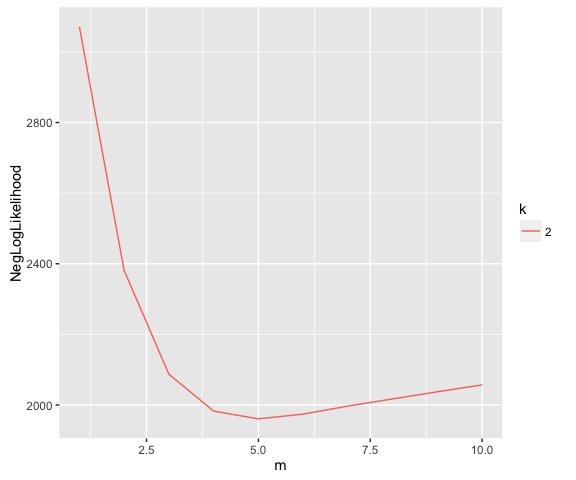It looks like the optimal m is 5, which we can use to fit with all the data. We will also use the same m for the convex formulation.

logpca_model = logisticPCA(house_votes84, k = 2, m = which.min(logpca_cv))
clogpca_model = convexLogisticPCA(house_votes84, k = 2, m = which.min(logpca_cv))

Each of the formulations has a plot method to make it easier to see the results of the fit and assess convergence. There are three options for the type of plot. The first is type = "trace", which plots the deviance as a function of iteration. For logistic PCA and logistic SVD, the deviance should decrease at each iteration, but not necessarily for convex logistic PCA. For example, convex logistic PCA converged in 12 iterations.

plot(clogpca_model, type = "trace")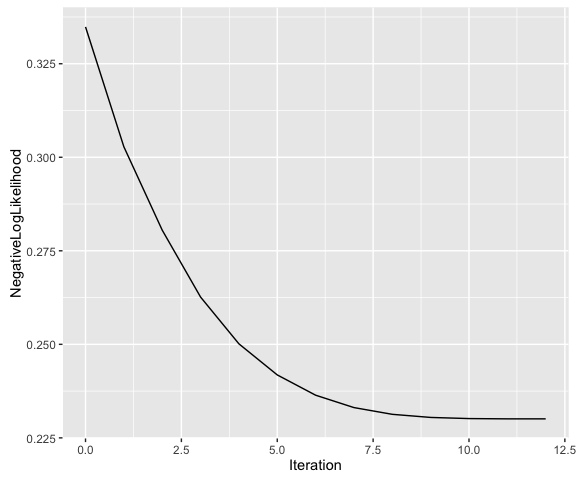In contrast, logistic SVD takes 549 iterations to converge.

plot(logsvd_model, type = "trace")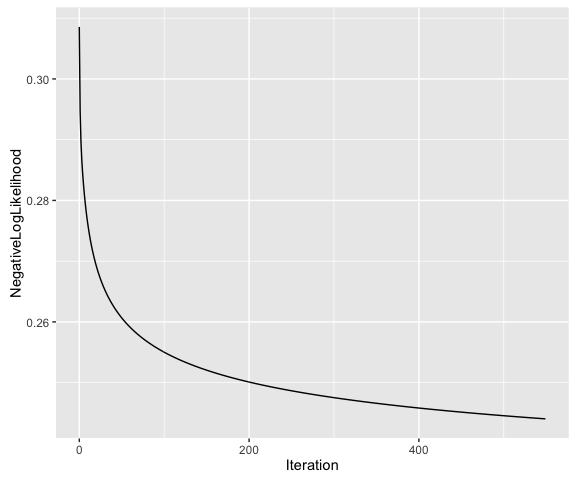With these formulations, each member of congress is approximated in a two-dimensional latent space. Below, we look at the PC scores for the congressmen, colored by their political party. All three formulations do a good job of separating the political parties based on voting record alone.

party = rownames(house_votes84)
plot(logsvd_model, type = "scores") + geom_point(aes(colour = party)) +
ggtitle("Exponential Family PCA") + scale_colour_manual(values = c("blue", "red"))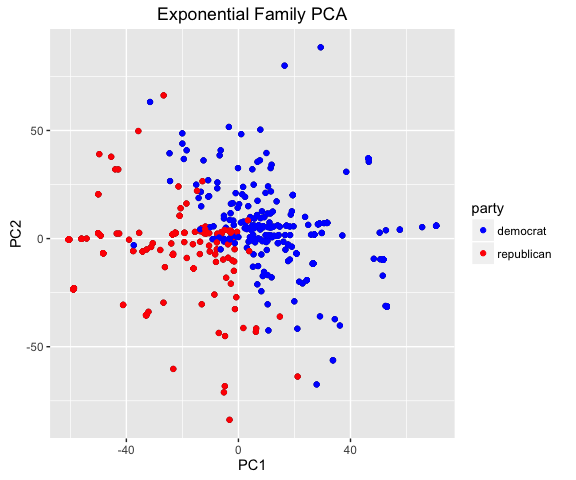plot(logpca_model, type = "scores") + geom_point(aes(colour = party)) +
ggtitle("Logistic PCA") + scale_colour_manual(values = c("blue", "red"))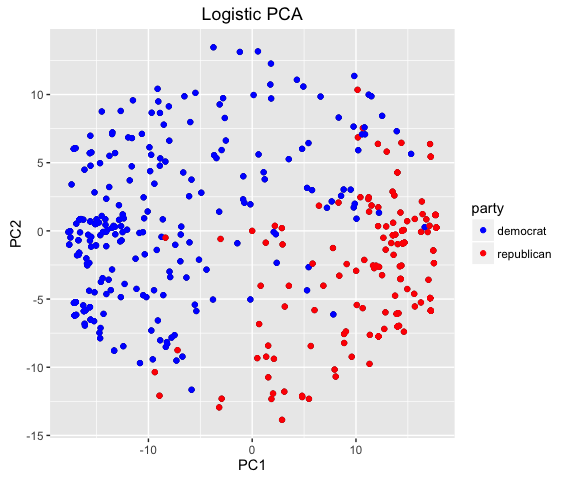plot(clogpca_model, type = "scores") + geom_point(aes(colour = party)) +
ggtitle("Convex Logistic PCA") + scale_colour_manual(values = c("blue", "red"))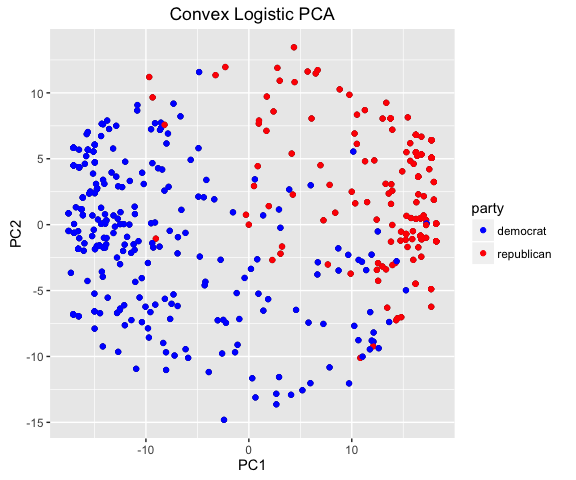For convex logistic PCA, we do not necessarily get a two-dimensional space with the Fantope matrix H. However, we use the first $$k$$ eigenvectors of H as an estimate of U.

One can also examine the latent space of the variables by using type = "loadings".

### Fitted and Predicted Estimates

The fitted function provides fitted values of either probabilities or natural parameters. For example,

head(fitted(logpca_model, type = "response"))
##           [,1]      [,2]       [,3]        [,4]      [,5]      [,6]
## [1,] 0.1575019 0.9075000 0.09229721 0.959218468 0.9986316 0.9982951
## [2,] 0.1437479 0.8202556 0.07417629 0.973745875 0.9986520 0.9980874
## [3,] 0.3432904 0.9997140 0.51135413 0.254949046 0.9967767 0.9988433
## [4,] 0.5858706 0.9998458 0.92339249 0.008711673 0.9159592 0.9903378
## [5,] 0.4657483 0.9995484 0.79074232 0.052783059 0.9742113 0.9949505
## [6,] 0.3798881 0.9904405 0.63830758 0.206453209 0.9679469 0.9903685
##             [,7]       [,8]        [,9]     [,10]     [,11]      [,12]
## [1,] 0.019043928 0.01432709 0.011477307 0.4792791 0.3316243 0.91157574
## [2,] 0.024706395 0.01455433 0.013016539 0.5094768 0.2002938 0.93258935
## [3,] 0.004112127 0.02379132 0.007081943 0.2611630 0.9913548 0.39722843
## [4,] 0.029769976 0.29774883 0.055012835 0.2193926 0.9979127 0.05401718
## [5,] 0.019372131 0.13154573 0.030119049 0.2589835 0.9925267 0.16240789
## [6,] 0.064734180 0.17096413 0.058714933 0.3591397 0.9028277 0.34250135
##          [,13]     [,14]      [,15]     [,16]
## [1,] 0.9602252 0.9995889 0.04969826 0.4417728
## [2,] 0.9588701 0.9997380 0.04567344 0.4533561
## [3,] 0.9567721 0.9714899 0.12396075 0.5084981
## [4,] 0.8224117 0.3859787 0.38413493 0.9433503
## [5,] 0.8877036 0.8173755 0.24711454 0.8636518
## [6,] 0.8571757 0.9521942 0.20613266 0.9066621

This could be useful if some of the binary observations are missing, which in fact this dataset has a lot of. The fitted probabilities give an estimate of the true value.

Finally, suppose after fitting the data, the voting record of a new congressman appears. The predict function provides predicted probabilities or natural parameters for that new congressman, based on the previously fit model and the new data. In addition, there is an option to predict the PC scores on the new data. This may be useful if the low-dimensional scores are inputs to some other model.

For example, let’s make up five fake congressmen.

d = ncol(house_votes84)
votes_fake = matrix(sample(c(0, 1), 5 * d, replace = TRUE), 5, d,
dimnames = list(NULL, colnames(house_votes84)))

Now, we can use the models we fit before to estimate the PC scores of these congressmen in the low-dimensional space. One advantage of logistic PCA is that it is very quick to estimate these scores on new observations, whereas logistic SVD must solve for A on the new data.

predict(logpca_model, votes_fake, type = "PCs")
##           [,1]       [,2]
## [1,] -1.152756  -1.776178
## [2,] -2.312936  -3.238975
## [3,] -0.199700 -11.052720
## [4,]  3.698878  -0.130449
## [5,]  5.040767  -5.915849